# 13- PROBABILITY

Here we are going through the ncert solutions for class 12 maths chapter 13 – probability. so before going through the ncert solutions make sure to go through the textbook that helps you to understand the solutions more easily.

# Exercise 13.1

#### Question 1:

Given that E and F are events such that P(E) = 0.6, P(F) = 0.3 and P(E ∩ F) = 0.2, find P (E|F) and P(F|E).

It is given​​ that P(E) = 0.6, P(F) = 0.3, and P(E ∩ F) = 0.2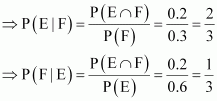#### Question 2:

Compute P(A|B), if P(B) = 0.5 and P (A ∩ B) = 0.32

It is given that P(B) = 0.5 and P(A ∩ B) = 0.32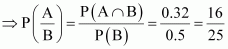#### Question 3:

If P(A) = 0.8, P(B) = 0.5 and P(B|A) = 0.4, find

(i) P(A ∩ B) (ii)​​ P(A|B) (iii) P(A​​ ​​ B)

It is given that P(A) = 0.8, P(B) = 0.5, and P(B|A) = 0.4

(i) P (B|A) = 0.4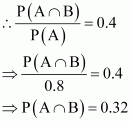(ii)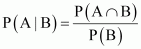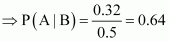(iii)

P(A∪B) = P(A) + P(B) − P(A∩B)⇒P(A∪B)=0.8 + 0.5 − 0.32 = 0.98PAB = PA + PB - PA∩BPAB=0.8 + 0.5 - 0.32 = 0.98

#### Question 4:

Evaluate P (A​​ ​​ B), if 2P (A) = P (B) =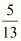and P(A|B) =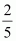It is given that,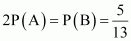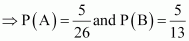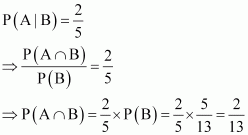It is known that,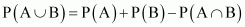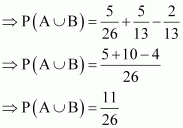#### Question 5:

If P(A)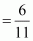, P(B) =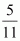and P(A​​ ​​ B) =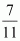, find

(i) P(A ∩ B) (ii) P(A|B) (iii) P(B|A)

It is​​ given that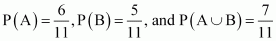(i)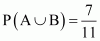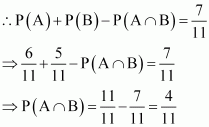(ii) It is known that,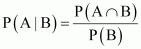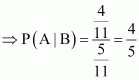(iii) It is known that,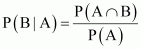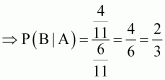#### Question 6:

A coin is tossed three times, where

(i) E: head on third toss, F: heads on first two tosses

(iii) E: at most two​​ tails, F: at least one tail

If a coin is tossed three times, then the sample space S is

S = {HHH, HHT, HTH, HTT, THH, THT, TTH, TTT}

It can be seen that the sample space has 8 elements.

(i) E = {HHH, HTH, THH, TTH}

F = {HHH, HHT}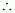E ∩ F =​​ {HHH}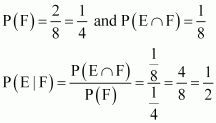(ii) E = {HHH, HHT, HTH, THH}

F = {HHT, HTH, HTT, THH, THT, TTH, TTT}E ∩ F = {HHT, HTH, THH}

Clearly,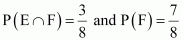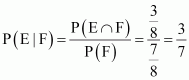(iii) E = {HHH, HHT, HTT, HTH, THH, THT, TTH}

F = {HHT, HTT, HTH, THH, THT, TTH, TTT}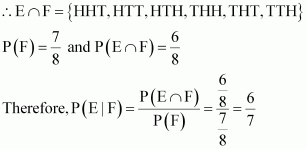Question 7:

Two coins are tossed once, where

(i)​​ E: tail appears on one coin, F: one coin shows head

(ii) E: not tail appears, F: no head appears

If two coins are tossed once, then the sample space S is

S = {HH, HT, TH, TT}

(i) E = {HT, TH}

F = {HT, TH}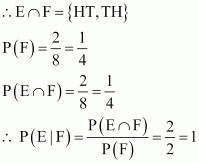(ii) E = {HH}

F = {TT}

​​ E ∩ F = Φ

P​​ (F) = 1 and P (E ∩ F) = 0

​​ P(E|F) =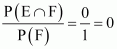#### Question 8:

A die is thrown three times,

E: 4 appears on the third toss, F: 6 and 5 appears respectively on first two tosses

If a die is thrown three times, then the number of elements in the sample space​​ will be 6 × 6 × 6 = 216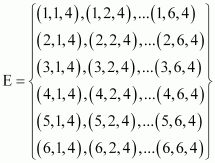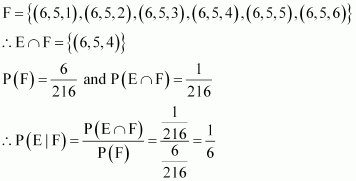#### Question 9:

Mother, father and son line up at random for a family picture

E: son on one end, F: father in middle

If mother (M), father (F), and son (S) line up for the family picture, then the sample space will be

S =​​ {MFS, MSF, FMS, FSM, SMF, SFM}

​​ E = {MFS, FMS, SMF, SFM}

F = {MFS, SFM}

​​ E ∩ F = {MFS, SFM}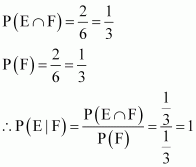#### Question 10:

A black and a red dice are rolled.

(a) Find the conditional probability of obtaining a sum greater than 9, given that the black die​​ resulted in a 5.

(b) Find the conditional probability of obtaining the sum 8, given that the red die resulted in a number less than 4.

Let the first observation be from the black die and second from the red die.

When two dice (one black and another​​ red) are rolled, the sample space S has 6 × 6 = 36 number of elements.

• Let

A: Obtaining a sum greater than 9

= {(4, 6), (5, 5), (5, 6), (6, 4), (6, 5), (6, 6)}

B: Black die results in a 5.

= {(5, 1), (5, 2), (5, 3), (5, 4), (5, 5), (5, 6)}

​​ A ∩ B = {(5,​​ 5), (5, 6)}

The conditional probability of obtaining a sum greater than 9, given that the black die resulted in a 5, is given by P (A|B).

P(A|B) = P(A∩B)P(B) = 236636 = 26 = 13PA|B = PA∩BPB = 236636 = 26 = 13

(b) E: Sum of the observations is 8.

= {(2,​​ 6), (3, 5), (4, 4), (5, 3), (6, 2)}

F: Red die resulted in a number less than 4.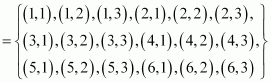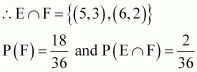The conditional probability of obtaining the sum equal to 8, given that the red die resulted in a number less than 4, is given by P (E|F).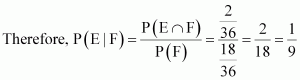#### Question 11:

A fair die is​​ rolled. Consider events E = {1, 3, 5}, F = {2, 3} and G = {2, 3, 4, 5}

Find

(i) P (E|F) and P (F|E) (ii) P (E|G) and P (G|E)

(ii) P ((E​​ ​​ F)|G) and P ((E ∩ G)|G)

When a fair die is rolled, the sample space S will be

S = {1, 2, 3, 4, 5, 6}

It is given that E = {1, 3, 5}, F = {2, 3}, and G = {2, 3, 4, 5}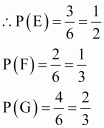(i) E ∩ F = {3}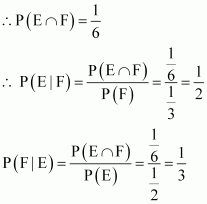(ii) E ∩ G = {3, 5}